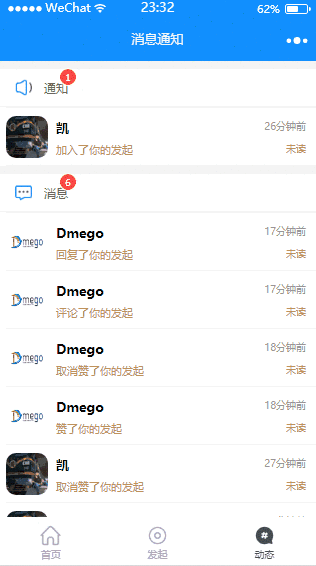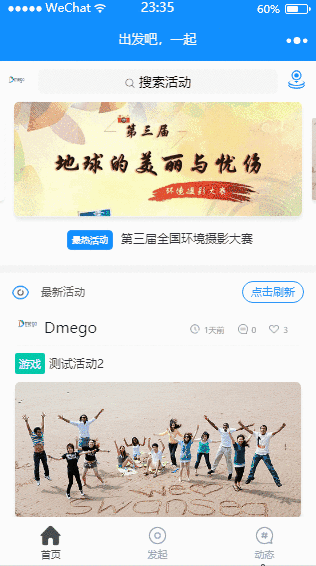#0
0
01. 云栖社区>
2. 博客>
3. 正文

## 微信小程序开发问题汇总

dmego 2017-12-04 15:57:00 浏览1160## 小程序简介

“让兴趣不再孤单，让爱好不再流浪” 是微信小程序《出发吧一起》的主题，这款小程序旨在解决当代大学生在校园生活中的孤独感，让大家找到志同道合的朋友，在跑步、健身、竞赛等活动中找到伙伴。利用小程序即开即用，用完就走的特点与交友相结合，它将会是一款高效快捷、无负担的线下交友利器

## 开发中技术问题汇总

### 1.使用e.target.dataset的出现问题

• WXML
``<view bindtap="bintap" data-id="1"></view>``
• JS
``````bintap:function(e){
var id = e.currentTarget.dataset.id;
}``````

### 2.小程序 textarea 文本框如何显示实时字数

• WXML
``````<view>
<view>
<textarea name="content" bindinput="bindTextAreaChange" maxlength="{{noteMaxLen}}" />
<view class="chnumber">{{noteNowLen}}/{{noteMaxLen}}</view>
</view>
</view>``````
• JS
``````data:{
noteMaxLen: 200,//备注最多字数
noteNowLen: 0,//备注当前字数
}

//字数改变触发事件
bindTextAreaChange: function (e) {
var that = this
var value = e.detail.value,
len = parseInt(value.length);
if (len > that.data.noteMaxLen)
return;
that.setData({
content: value, noteNowLen: len
})
},``````

### 3.利用 JS 实现模糊查询

• JS
``````//js 实现模糊匹配查询
findEach: function (e) {
var that = this
var strFind = that.data.wxSearchData.value; //这里使用的 wxSearch 搜索UI插件,
if (strFind == null || strFind == "") {
wx.showToast({
title: '输入为空',
})
}
if (strFind != "") {
var nPos;
var resultPost = [];
for (var i in smoodList) {
var sTxt = smoodList[i].title || ''; //活动的标题
nPos = sTxt.indexOf(strFind);
if (nPos >= 0) {//如果输入的关键字在该活动标题中出现过,则匹配该活动
resultPost.push(smoodList[i]); //将该活动加入到搜索到的活动列表中
}
}
that.setData({
moodList: resultPost
})
}
},``````

### 4.使用 JS 将字符串格式的时间转换成几秒前,几分钟前...

• JS
``````//字符串转换为时间戳
function getDateTimeStamp(dateStr) {
return Date.parse(dateStr.replace(/-/gi, "/"));
}
//格式化时间
function getDateDiff(dateStr) {
var publishTime = getDateTimeStamp(dateStr) / 1000,
d_seconds,
d_minutes,
d_hours,
d_days,
timeNow = parseInt(new Date().getTime() / 1000),
d,

date = new Date(publishTime * 1000),
Y = date.getFullYear(),
M = date.getMonth() + 1,
D = date.getDate(),
H = date.getHours(),
m = date.getMinutes(),
s = date.getSeconds();
//小于10的在前面补0
if (M < 10) {
M = '0' + M;
}
if (D < 10) {
D = '0' + D;
}
if (H < 10) {
H = '0' + H;
}
if (m < 10) {
m = '0' + m;
}
if (s < 10) {
s = '0' + s;
}

d = timeNow - publishTime;
d_days = parseInt(d / 86400);
d_hours = parseInt(d / 3600);
d_minutes = parseInt(d / 60);
d_seconds = parseInt(d);

if (d_days > 0 && d_days < 3) {
return d_days + '天前';
} else if (d_days <= 0 && d_hours > 0) {
return d_hours + '小时前';
} else if (d_hours <= 0 && d_minutes > 0) {
return d_minutes + '分钟前';
} else if (d_seconds < 60) {
if (d_seconds <= 0) {
return '刚刚';
} else {
return d_seconds + '秒前';
}
} else if (d_days >= 3 && d_days < 30) {
return M + '-' + D + ' ' + H + ':' + m;
} else if (d_days >= 30) {
return Y + '-' + M + '-' + D + ' ' + H + ':' + m;
}
}``````

### 5.微信小程序提交表单清空表单数据

• WXML
``````<form bindsubmit="submitForm">
<text class="key">活动名称</text>
<input name="title"  maxlength="100" value="{{title}}" />
<button  formType="submit">确定</button>
</form>``````
• JS
``````submitForm:function(e){
var title = e.detail.value.title;
......
success: function (res) {
//将title值设置空
that.setData({
title: ''
}
}
}``````

### 6.微信号,QQ号,手机号 正则校验

• JS
``````    var wxReg = new RegExp("^[a-zA-Z]([-_a-zA-Z0-9]{5,19})+\$"); //微信号正则校验
var qqReg = new RegExp("[1-9][0-9]{4,}"); //QQ号正则校验
var phReg = /^1\d{9}\$/; //手机号正则校验
var nameReg = new RegExp("^[\u4e00-\u9fa5]{2,4}\$"); //2-4位中文姓名正则校验``````

### 7.使用 Bmob SDK 实现报名成功发送模板消息,生成小程序二维码等

,就是在小程序发布后 模板消息中如果带有 `page` 参数将不会发送,但是在开发版中能发送成功, 这个问题已经反馈了,估计等Bmob小程序`SDK`更新后会解决这个问题.

## 截图 & GIF## Bmob 数据库表结构设计

#### 用户表:(_User，自带表)

``````|--objectId //Id
|--userPic(String) //用户头像
|--nickname(String) //昵称
|--sex(Number) //性别
|--userData(Object) //微信登录用户数据
|--eventJoin(Array) //参加的活动Id 数组Array
|--eventFavo(Array) //收藏的活动Id 数组Array
|--feednum(Number) //反馈次数``````

#### 活动信息表：(Events)

``````|--objectId //活动Id
|--publisher(Pointer-->_User) //发起人
|--title(String) //活动主题
|--content(String) //活动内容
|--actpic(File) //活动宣传照片
|--acttype(String) //活动类别
{
1：运动,2：游戏,3：交友,
4：旅行,5：读书,6：竞赛,
7：电影,8: 音乐,9: 其他
}
|--isShow(Number) //是否公开显示在首页
|--endtime(String) //组队截止时间
|--latitude(Number)  //地址纬度
|--longitude(Number) //地址经度
|--peoplenum(String)//人数限制
|--likenum(Number)  //点赞数
|--liker(Array) //点赞人Id集合
|--commentnum(Number) //评论数
|--joinnumber(Number) // 现在参加的人数
|--joinArray(Array) // 现在参加的人集合``````

#### 活动信息扩展表:(EventMore)

``````|--objectId //活动信息扩展表Id
|--event(Pointer-->Events) //活动
|--Status(Number) //活动状态,(1:准备中,2:进行中,3:已结束)
|--Statusname(String) //活动状态名称
|--qrcode(File) //活动群聊二维码``````

``````|--objectId //评论Id
|--publisher(Pointer-->_User) //评论发布者
|--event(Pointer-->Events) //评论的活动
|--content(String)  //评论内容``````

#### 点赞表：(Likes)

``````|--objectId //点赞的Id
|--liker(Pointer-->_User) //点赞人
|--event(Pointer-->Events) //点赞的活动``````

#### 收藏表:(Favos)

``````|--objectId //收藏的Id
|--favor(Pointer-->_User)  //收藏者
|--event(Pointer-->Events) //收藏的活动``````

#### 消息通知表:(Plyre)

``````|--objectId //消息通知的Id
|--fid(String)  //活动发布者Id(被赞或者被取消赞的人的ID,或者被回复,被评论的人的ID)(被通知的人)
|--uid(Pointer-->_User)   //消息通知人
|--wid (String) //被赞，或者取消赞，被评论，或者被回复，加入，取消加入的活动id
|--avatar (String) //消息通知人的头像
|--bigtype(Number) //消息通知大类(1代表消息，2代表通知)
|--behavior(Number) //(消息提醒类型)
{
1：赞
2：取消赞
3：被评论
4：被回复
5：加入活动
6：取消加入活动
7：修改了加入信息
}``````

#### 活动联系表:(Contact)

``````|--objectId //联系表Id
|--publisher(Pointer-->_User)  //活动发布者
|--currentUser (Pointer-->_User) //当前用户
|--event(Pointer-->Events)  //想要加入的活动
|--realname (String) //真实姓名
|--contactWay(String) //联系方式(微信号,手机号,QQ号)
|--contactValue(String) //联系方式的号码``````

#### 反馈表:(Feedback)

``````|--objectId //反馈Id
|--feedUser(Pointer-->_User) //反馈人Id
|--title(String) //反馈标题
|--content(String) //反馈内容
|--feedpic(File) //反馈图片
|--feedinfo(String) //反馈用户的设备信息     ``````

.....

dmego
+ 关注